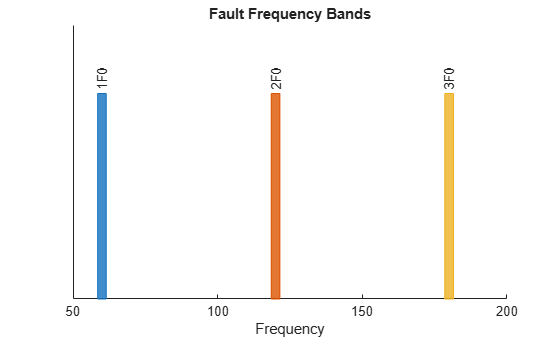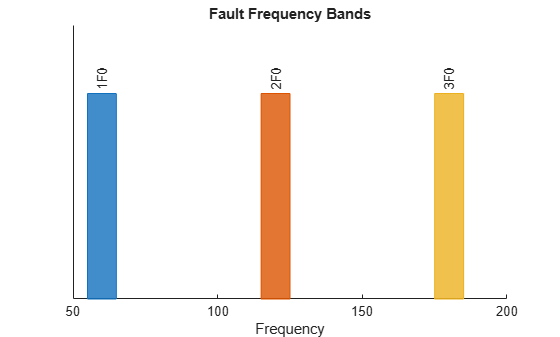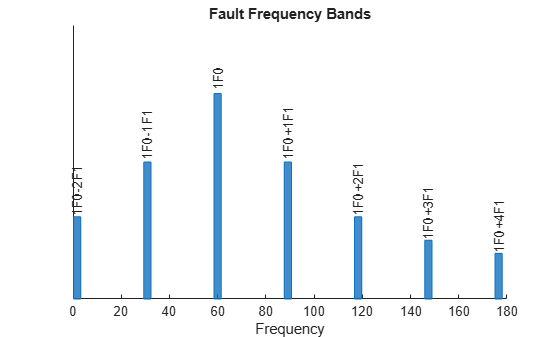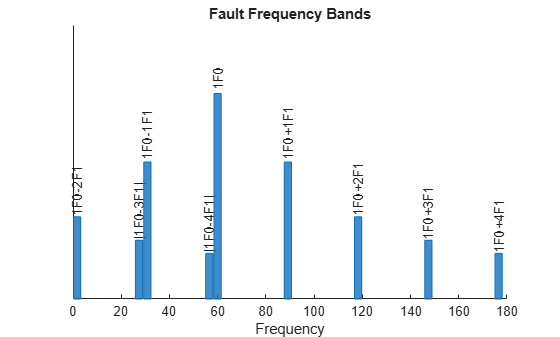Documentation

# faultBands

Generate fault frequency bands for spectral feature extraction

## Syntax

``FB = faultBands(F0,N0)``
``FB = faultBands(F0,N0,F1,N1)``
``___ = faultBands(___,Name,Value)``
``[FB,info] = faultBands(___)``
``faultBands(___)``

## Description

example

````FB = faultBands(F0,N0)` generates fault frequency bands `FB`, using the fundamental frequency of interest `F0` and the array of harmonics `N0`. For instance, to construct fault bands for an induction motor, the mains frequency of 60 Hz is the fundamental frequency of interest.```

example

````FB = faultBands(F0,N0,F1,N1)` constructs fault frequency bands `FB`, using the distance of the first sideband `F1` from the fundamental frequency `F0`. `N1` is the array of the sidebands around `F0`. If `F1` is not specified, then `faultBands` sets `F1` to 10 percent of `F0` by default. `N1` is equivalent to the '`Sidebands`' name-value pair. You can use the '`Type`' name-value pair to specify separation between successive sidebands.```

example

````___ = faultBands(___,Name,Value)` allows you to specify additional parameters using one or more name-value pair arguments.```

example

````[FB,info] = faultBands(___)` also returns the structure `info` containing information about the generated fault frequency bands `FB`.```

example

````faultBands(___)` with no output arguments plots a bar chart of the generated fault frequency bands `FB`.```

## Examples

collapse all

For this example, generate frequency bands for analyzing the signal components around the first 5 harmonics of the mains supply frequency.

With the fundamental frequency of 60 Hz, the frequency of the alternating current in the mains power supply, use `faultBands` to generate the first 5 harmonics of the mains supply.

```F0 = 60; N0 = 1:5; FB = faultBands(F0,N0)```
```FB = 5×2 58.5000 61.5000 118.5000 121.5000 178.5000 181.5000 238.5000 241.5000 298.5000 301.5000 ```

`FB` is returned as a 5x2 array with default frequency band width of 5% of `F0` which is 3 Hz. The first column in `FB` contains the values of $F-\frac{W}{2}$, while the second column contains all the values of $F+\frac{W}{2}$ for each harmonic.

For this example, consider an induction motor with broken rotor bars. Under normal operation with load, the rotor speed always lags the speed of the magnetic field allowing the rotor bars to cut magnetic lines of force and produce useful torque. This difference is called slip. Considering a slip value of 0.03 in the system with broken rotors, construct frequency bands for sideband components around the fundamental frequency of 60 Hz.

```F0 = 60; N0 = 1:2; slip = 0.03; F1 = 2*slip*F0; N1 = 1:3; [FB,info] = faultBands(F0,N0,F1,N1)```
```FB = 12×2 47.7000 50.7000 51.3000 54.3000 54.9000 57.9000 62.1000 65.1000 65.7000 68.7000 69.3000 72.3000 107.7000 110.7000 111.3000 114.3000 114.9000 117.9000 122.1000 125.1000 ⋮ ```
```info = struct with fields: Centers: [1x12 double] Labels: [1x12 string] HarmonicGroups: [1 1 1 1 1 1 2 2 2 2 2 2] ```

Construct frequency bands for analyzing the signal components around the first three harmonics of the electrical mains supply frequency.

With the fundamental frequency of 60 Hz, the alternating current in the mains power supply, use `faultBands` to visualize the first 3 harmonics of the mains supply.

```F0 = 60; N0 = 1:3; faultBands(F0,N0)```From the plot, observe the following:

• The fundamental frequency, which is also the first harmonic, `1F0` at 60 Hz

• The second harmonic, `2F0` at 120 Hz

• The third harmonic, `3F0` at 180 Hz

To better capture the expected variations of the actual system signals around the nominal fault frequencies, set the widths of each band to 10 Hz.

`faultBands(F0,N0,'Width',10)`For this example, consider an induction motor with static and dynamic rotor eccentricities. Construct and visualize the frequency bands for the 4 sideband components of an induction motor with 4 pole pairs around the fundamental frequency due to the rotor eccentricities.

```F0 = 60; N0 = 1; slip = 0.029; polePairs = 4; F1 = 2*F0*(1-slip)/polePairs```
```F1 = 29.1300 ```
```N1 = 0:4; faultBands(F0,N0,F1,N1)```
```Warning: Truncated or removed negative fault frequency bands. ```To avoid truncating negative fault frequency bands, set '`Folding`' to `true` to fold them onto the positive frequency axis.

`faultBands(F0,N0,F1,N1,'Folding',true)`Observe that the sideband frequencies `1F0-3F1` and `1F0-4F1` are now visible on the positive axis.

## Input Arguments

collapse all

Fundamental frequency of interest, specified as a positive scalar. `faultBands` constructs the fault frequency bands around the fundamental frequency `F0`. For instance, to construct fault bands for a faulty induction motor, the mains frequency of 60 Hz is the fundamental frequency of interest. Similarly, to generate fault bands for a faulty gear train, the input shaft frequency is the fundamental frequency.

You can specify `F0` in either hertz or orders.

Harmonics of the fundamental frequency, specified as a vector of positive integers. Specify fault bands around the fundamental frequency `F0` and its harmonics by `N0`. `N0` is equivalent to the '`Harmonics`' name-value pair with a default value of 1.

Distance of the first sideband from the fundamental frequency, specified as a positive scalar. If `F1` is not specified, then `faultBands` assumes a value of 10 percent of the fundamental frequency for `F1`.

Sidebands of the fundamental frequency and its harmonics, specified as a vector of nonnegative integers. `N1` is equivalent to the '`Sidebands`' name-value pair with a default value of 0.

### Name-Value Pair Arguments

Specify optional comma-separated pairs of `Name,Value` arguments. `Name` is the argument name and `Value` is the corresponding value. `Name` must appear inside quotes. You can specify several name and value pair arguments in any order as `Name1,Value1,...,NameN,ValueN`.

Example: `...,'Harmonics',[1,3,5]`

Harmonics of the fundamental frequency to be included, specified as the comma-separated pair consisting of '`Harmonics`' and a vector of positive integers. The default value is 1. Specify '`Harmonics`' when you want to construct the frequency bands with more harmonics of the fundamental frequency.

Sidebands around the fundamental frequency and its harmonics to be included, specified as the comma-separated pair consisting of '`Sidebands`' and a vector of nonnegative integers. The default value is 0. Specify '`Sidebands`' when you want to construct the frequency bands with sidebands around the fundamental frequency and its harmonics.

Width of the frequency bands centered at the nominal fault frequencies, specified as the comma-separated pair consisting of '`Width`' and a positive scalar. The default value is 5 percent of the fundamental frequency. Avoid specifying '`Width`' with a large value so that the fault bands do not overlap.

Separation value between successive sidebands, specified as the comma-separated pair consisting of '`Type`' and either `'additive'` or `'multiplicative'`. Specify '`Type`' as:

• `'additive'`, to set the separation between successive sidebands to `F1`.

• `'multiplicative'`, to set the separation between successive sidebands proportional to both the harmonic order and the sideband value.

Logical value specifying whether negative nominal fault frequencies have to be folded about the frequency origin, specified as the comma-separated pair consisting of '`Folding`' and either `true` or `false`. If you set '`Folding`' to `true`, then `faultBands` folds the negative nominal fault frequencies about the frequency origin by taking their absolute values such that the folded fault bands always fall in the positive frequency intervals. The folded fault bands are computed as , where `W` is the '`Width`' name-value pair and `F` is one of the nominal fault frequencies.

## Output Arguments

collapse all

Fault frequency bands, returned as an `Nx2` array, where `N` is the number of fault frequencies. `FB` is returned in the same units as `F0`, in either Hertz or orders. The generated fault bands, , are centered depending on the sideband specification as follows:

• If you do not specify the sidebands, then the fault bands are centered at $F={n}_{0}{F}_{0}$, where the integer `n0` ranges through the elements of the array of harmonics, `N0`.

• If you specify sidebands using `N1` or the '`Sidebands`' name-value pair, then fault bands are centered at:

• $F={n}_{0}{F}_{0}±{n}_{1}{F}_{1}$, when '`Type`' is specified as `'additive'`. Here, the integer `n1` ranges through the elements of the array of sidebands, `N1`.

• $F={n}_{0}\left({F}_{0}±{n}_{1}{F}_{1}\right)$, when '`Type`' is specified as `'multiplicative'`.

Information about the fault frequency bands in `FB`, returned as a structure with the following fields:

• `Centers` — Center fault frequencies

• `Labels` — Labels describing each frequency

• `HarmonicGroups` — Harmonic group numbers equal to the harmonic order of each frequency band to be able to identify fault bands associated with the nominal fault frequency $F={n}_{0}{F}_{0}$, where the integer `n0` ranges through the elements of the array of harmonics, `N0`